# Gamma Stirling Engine

This example shows how to model a Gamma Stirling engine using gas, thermal, and mechanical Simscape™ components and domains.

Stirling engines absorb heat from an external source to partially transform it into mechanical power, and dissipate the rest in a cold thermal sink. The external heat source is the key difference with internal combustion engines, which produce heat from combustion reactions in the gas inside the system. In Stirling engines the gas is inert (for example, air, in this case).

### Model overview

The most typical designs of Stirling engines are alpha, beta and gamma configurations. In this example we only model the gamma configuration, which consists of two pistons connected with a passage pipe.

The first piston is called Displacer, which is a double-acting cylinder with two chambers, one is the heater, absorbing heat from a flame, and the other is the cooler, dissipating heat to the ambient. The overall volume of the displacer piston is constant, although gas flows from the cooler to the heater and vice-versa as the piston head moves. The flow between them is through the so-called Regenerator. The Regenerator is a pipe that allows flow between cooler and heater in the displacement piston. It is normally implemented as a piston head with smaller radius than the cylinder, allowing leakage.

The second piston is called Power piston, and is a single-acting cylinder with variable volume connected to the displacer through a passage pipe. This piston produces the torque and power.

Both displacer and power pistons are connected through two slider-crank mechanisms to a flywheel. The displacer crank has a 90 degree delay from the power piston.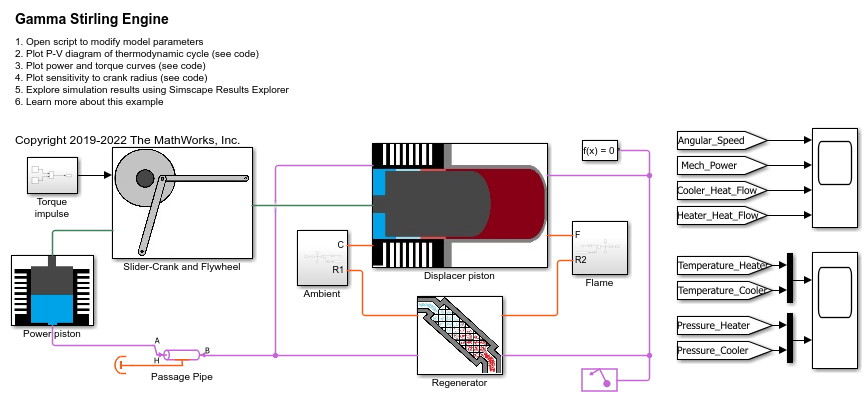The displacer piston: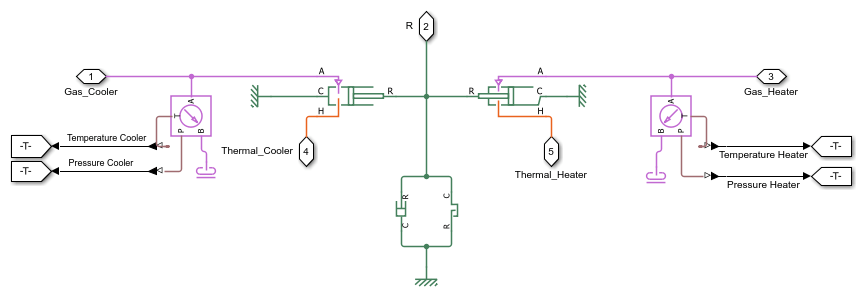The regenerator:The regenerator also conducts heat from the heater to the cooler.

The power piston: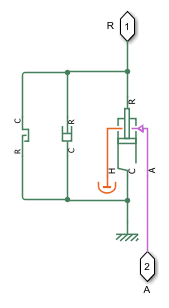The slider-cranks and flywheel:The user can choose to start the engine with a torque impulse and let it accelerate until steady-state, or force an angular speed, by commenting and uncommenting the torque source and the angular speed source.

The flame and ambient subsystems contain temperature sources and heat convection.

### Parameterization

Most parameters in the Simscape™ blocks of this example have been stored as variables in the script GammaStirlingEngineParams for easy modification. Edit the script to change parameter values.

### Simulation results

The model simulates 15s of Stirling engine start-up, by applying an impulse at t = 5s to set the flywheel in initial motion.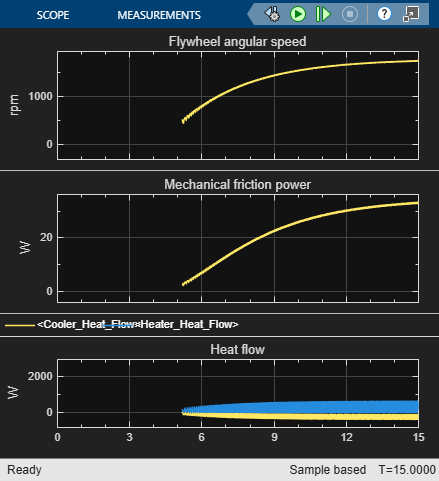### P-V diagram of thermodynamic cycle

A key graph to consider in engine design is the P-V diagram of the thermodynamic cycle. It plots gas pressure and volume in the power piston during a revolution of the flywheel. In steady-state, this curve is closed and cyclical. The area enclosed by the curve is the mechanical work provided during one cycle. The total area under the curve is the heat absorbed during one cycle. The ratio between the two is the thermodynamic efficiency of the cycle. If we multiply work per cycle (or heat per cycle) with the number of cycles per second, we obtain the mechanical power (or the heat power absorbed)

```Work per cycle: 1.3437J Heat absorption per cycle: 11.5156J Thermodynamic efficiency: 11.67% Mechanical power: 38.6874W Thermal power absorbed: 331.5646W ----------------------- ```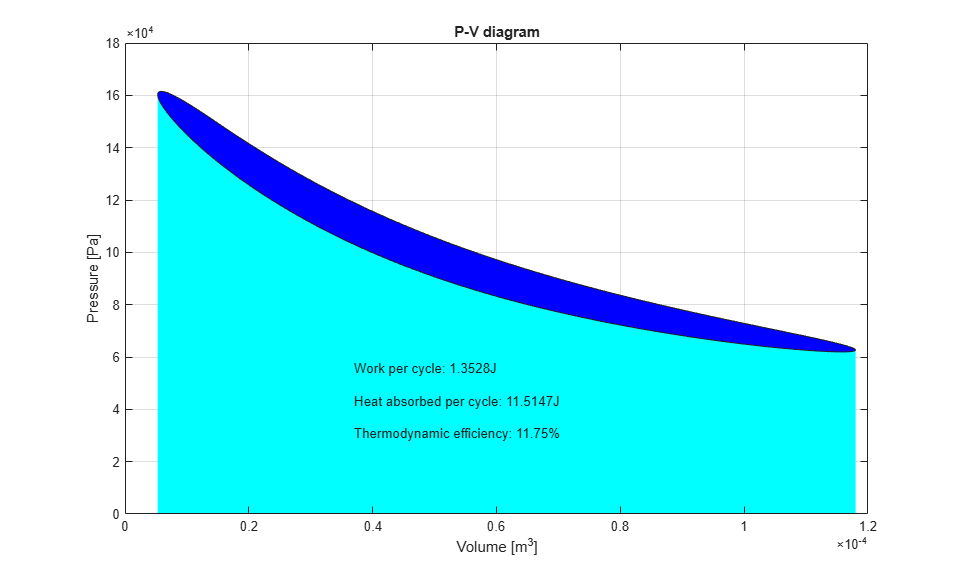### Power and torque curves

Another key performance indicator is the power-rpm curve and torque-rpm curve.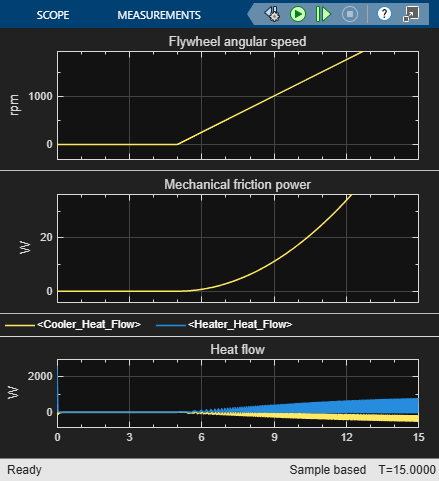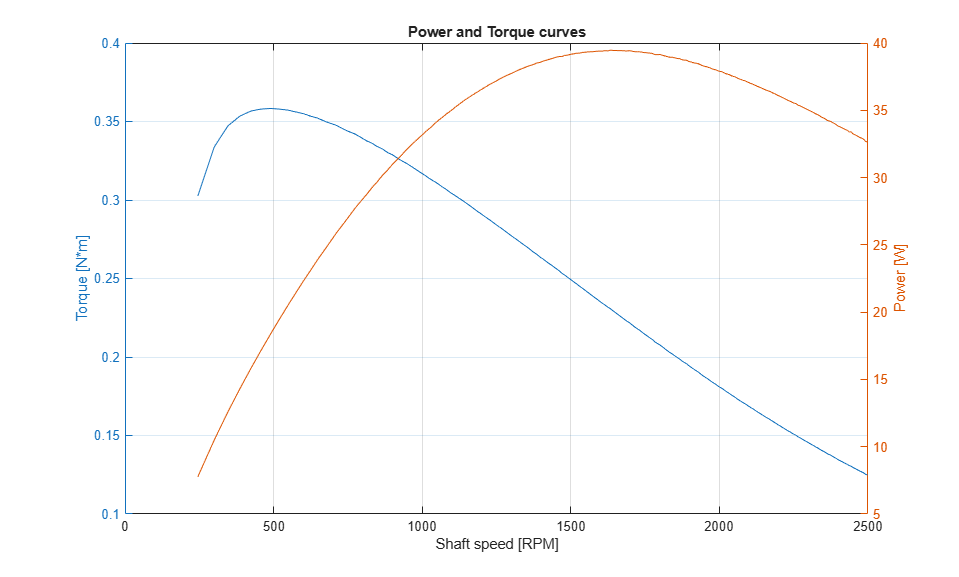### Design optimization

A great advantage of having a parameterized physical model is that optimization algorithms can be used to find optimal design parameters (for maximum efficiency or power). One of the possible design variables to optimize is power piston crank radius. In this section two values of power piston crank radius will be compared.

```1st crank radius Work per cycle: 1.3437J Heat absorption per cycle: 11.5156J Thermodynamic efficiency: 11.67% Shaft speed: 1727.5628rpm Mechanical power: 38.6874W Thermal power absorbed: 331.5646W ----------------------- 2nd crank radius Work per cycle: 1.2831J Heat absorption per cycle: 8.1104J Thermodynamic efficiency: 15.82% Shaft speed: 1613.6364rpm Mechanical power: 34.5063W Thermal power absorbed: 218.12W ----------------------- ```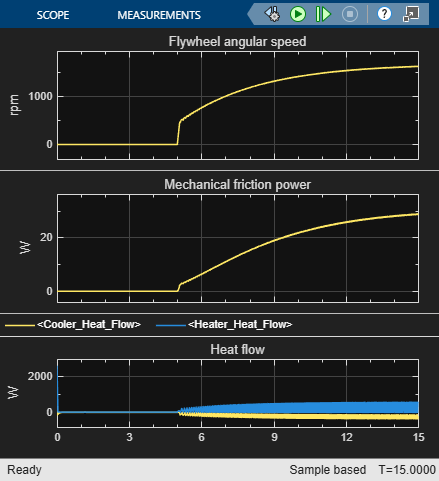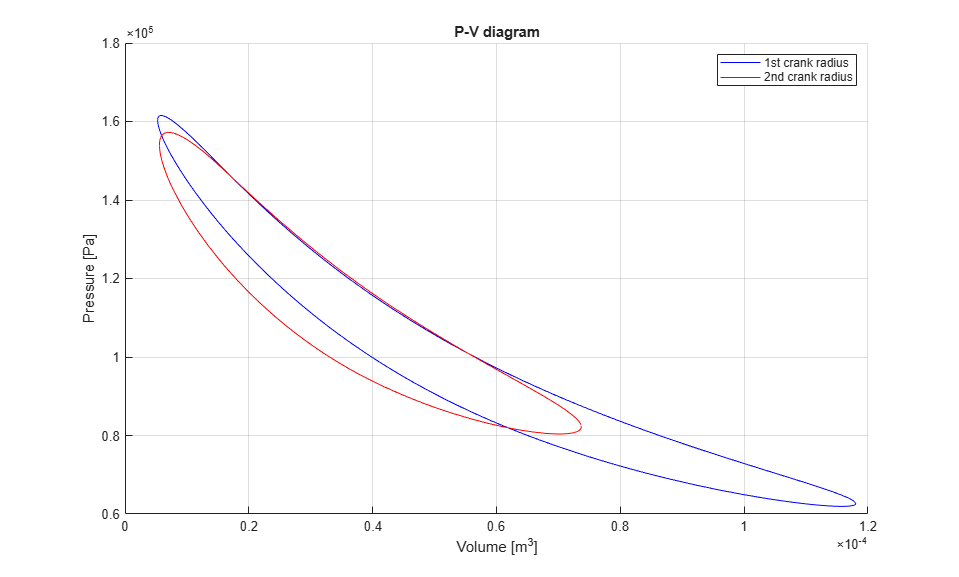With the second value of crank radius we obtain lower shaft speed and lower power, but higher thermodynamic efficiency. This approach could be used in a multi-variable optimization process to find a global optimal design with genetic algorithms, for example.